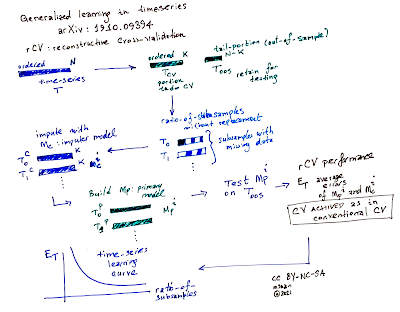## Tuesday, 22 June 2021

### Full cross-validation and generating learning curves for time-series models

Kindly reposted to KDnuggets by Gregory Piatetsky-Shapiro

## Preamble

Time-series analysis is needed almost in any quantitative field and real-life systems that collects data over time, i.e., temporal datasets. Building predictive models on temporal datasets for future evolution of systems in consideration are usually called forecasting. The validation of such models deviates from the standard holdout method of having random disjoint splits of train, test and validation sets used in supervised learning. This stems from the fact that time-series are ordered and order induces all sorts of statistical properties that should be retained. For this reason, applying direct cross-validation to time-series model building is not possible and only restricted to out-of-sample (OOS) validation, using the end-tail of a temporal set as a single test set. A recent work proposed an approach that overcomes the known limitation achieving full cross-validation for time-series. The approach opens up for a possibility to produce learning curves for the time-series models as well, which is usually also not possible due to similar reasons.

## Reconstructive Cross-validation (rCV) : A meta-algorithm design principles

rCV is proposed recently in the paper titled Generalised learning of time-series: Ornstein-Uhlenbeck processes. The design principles of rCV for time-series aims at the following principles:Figure 1 : rCV meta-algorithm for time-seriescross-validation and learning curves.

1. Logically close to standard cross-validation: Arbitrary test-set size and number of folds.
2. Preserve correlations and data order.
3. Does not create absurdity of predicting past from the future data.
4. Applicable in generic fashion regardless of learning algorithm.
5. Applicable to multi-dimensional time-series.
6. Evaluation metric agnostic.

Idea of introducing missing-data  : Temporal cross-validation and learning curves

The key idea of rCV is to create cross-validation sets via creating missing-data sets K-times, as in K-fold, with a given degree of missing ratio, i.e., random data point removal. Each fold will have disjoint set of missing data points. By an imputation method, we would fill out the K-disjoint missing data sets and generate K-different training datasets.  This would allow us to have K-different models and we could measure the generalised performance of the modelling approach by testing the primary models prediction on the Out-of-sample (OOS) test set. To avoid confusion about what is a model?, what we are trying to achieve is to find out hypothesis, i.e., the modelling approach.  By changing the ratio of missing data and repeating the cross-validation exercise will yield to set of ratio of missing-missing data introduced and their corresponding rCV errors, the plot is nothing but a learning-curve from supervised learning perspective.  Note that the imputation and prediction models are different models. The primary model we are trying to build is the prediction model we used for producing OOS predictions. The procedure is summarised in Figure 1.Figure 2 : Synthetic data and reconstructions.

## Show-case with Gaussian process models on Ornstein-Uhlenbeck processes

To demonstrate the utility of rCV, the mentioned paper uses a synthetic data generated by Ornstein-Uhlenbeck process, i.e., Gaussian process with certain parameter setting.  Figure 2, shows the synthetic data and example locations of generated missing-data sets's reconstruction errors. Figure 3 shows learning curves depending on the different ratios of missing data setting.Figure 3: Learning curves for the Gaussian Process modelgenerated by rCV.

Conclusion

rCV provides logically consistent way of practicing cross-validation in time-series. It is usually not possible to produce learning-curves on the same time-window for time-series model: by using rCV with different ratio missing data achieves this as well. rCV paves way to do generalised learning for time-series.